RESPONSE Statement
RESPONSE <function></ options> ;

The RESPONSE statement specifies functions of the response probabilities. The procedure models these response functions as linear combinations of the parameters.

By default, PROC CATMOD uses the standard response functions (generalized logits, which are explained in detail in the section Understanding the Standard Response Functions). With these standard response functions, the default estimation method is maximum likelihood, but you can use the WLS option in the MODEL statement to request weighted least squares estimation. With other response functions (specified in the RESPONSE statement), the default (and only) estimation method is weighted least squares.

You can specify more than one RESPONSE statement, in which case each RESPONSE statement produces a separate analysis. If the computed response functions for any population are linearly dependent (yielding a singular covariance matrix), then PROC CATMOD displays an error message and stops processing. See the section Cautions for methods of dealing with this.

The function specification can be any of the items in the following list. For an example of response functions generated and formulas for(the number of response functions), see the section More on Response Functions.

ALOGIT
ALOGITS

specifies response functions as adjacent-category logits of the marginal probabilities for each of the dependent variables. For each dependent variable, the response functions are a set of linearly independent adjacent-category logits, obtained by taking the logarithms of the ratios of two probabilities. The denominator of theth ratio is the marginal probability corresponding to theth level of the variable, and the numerator is the marginal probability corresponding to the (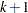)th level. If a dependent variable has two levels, then the adjacent-category logit is the negative of the generalized logit.

CLOGIT
CLOGITS

specifies that the response functions are cumulative logits of the marginal probabilities for each of the dependent variables. For each dependent variable, the response functions are a set of linearly independent cumulative logits, obtained by taking the logarithms of the ratios of two probabilities. The denominator of theth ratio is the cumulative probability,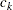, corresponding to theth level of the variable, and the numerator is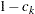(Agresti; 1984, 113–114). If a dependent variable has two levels, then PROC CATMOD computes its cumulative logit as the negative of its generalized logit. You should use cumulative logits only when the dependent variables are ordinally scaled.

JOINT

specifies that the response functions are the joint response probabilities. A linearly independent set is created by deleting the last response probability. For the case of one dependent variable, the JOINT and MARGINALS specifications are equivalent.

LOGIT
LOGITS

specifies that the response functions are generalized logits of the marginal probabilities for each of the dependent variables. For each dependent variable, the response functions are a set of linearly independent generalized logits, obtained by taking the logarithms of the ratios of two probabilities. The denominator of each ratio is the marginal probability corresponding to the last observed level of the variable, and the numerators are the marginal probabilities corresponding to each of the other levels. If there is one dependent variable, then specifying LOGIT is equivalent to using the standard response functions.

MARGINAL
MARGINALS

specifies that the response functions are marginal probabilities for each of the dependent variables in the MODEL statement. For each dependent variable, the response functions are a set of linearly independent marginals, obtained by deleting the marginal probability corresponding to the last level.

MEAN
MEANS

specifies that the response functions are the means of the dependent variables in the MODEL statement. This specification requires that all of the dependent variables be numeric.

specifies that the response functions and their covariance matrix are to be read directly from the input data set with one response function for each variable named. See the section Inputting Response Functions and Covariances Directly for more information.

transformation

specifies response functions that can be expressed by using successive applications of the four operations: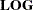,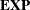,matrix literal, or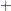matrix literal. The operations are described in detail in the section Using a Transformation to Specify Response Functions.

You can specify the following options in the RESPONSE statement after a slash.

OUT=SAS-data-set

produces a SAS data set that contains, for each population, the observed and predicted values of the response functions, their standard errors, and the residuals. Moreover, if you use the standard response functions, the data set also includes observed and predicted values of the cell frequencies or the cell probabilities. For further information, see the section Output Data Sets.

OUTEST=SAS-data-set

produces a SAS data set that contains the estimated parameter vector and its estimated covariance matrix. For further information, see the section Output Data Sets.

TITLE=’title’

displays the title at the top of certain pages of output that correspond to this RESPONSE statement.

### More on Response Functions

Suppose the dependent variable A has three levels and is the only response-effect in the MODEL statement. The following table shows the proportions upon which the response functions are defined:

 Value of A: Proportions: 1 2 3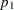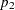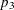Note that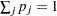. The following table shows the response functions generated for each population:

Function

Value

Specification

ofResponse Function

none2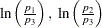ALOGITS

2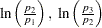CLOGITS

2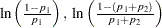JOINT

2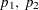LOGITS

2MARGINAL

2MEAN

1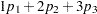Without a function specification, the default response functions are generalized logits.

Now, suppose the dependent variables A and B each have three levels (valued 1, 2, and 3 each) and the response-effect in the MODEL statement is A*B. The following table shows the proportions upon which the response functions are defined:

 Value of A: Value of B: Proportions: 1 1 1 2 2 2 3 3 3 1 2 3 1 2 3 1 2 3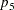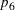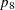The marginal totals for the preceding table are defined as follows: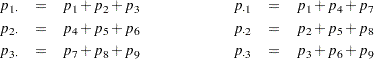where. The following table shows the response functions generated for each population:

Function

Value

Specification

ofResponse Function

none8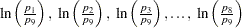ALOGITS

4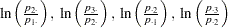CLOGITS

4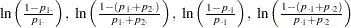JOINT

8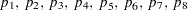LOGITS

4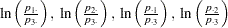MARGINAL

4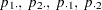MEAN

2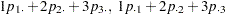Without a function specification, the default response functions are generalized logits.

The READ and transformation function specifications are not shown in the preceding table. For these two situations, there is not a general response function; the response functions that are generated depend on what you specify.

Another important aspect of the function specification is the number of response functions generated per population,. Let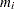represent the number of levels for theth dependent variable in the MODEL statement, and let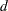represent the number of dependent variables in the MODEL statement. Then, if the function specification is ALOGITS, CLOGITS, LOGITS, or MARGINALS, the number of response functions is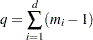If the function specification is JOINT or the default (generalized logits), the number of response functions per population is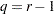whereis the number of response profiles. If every possible cross-classification of the dependent variables is observed in the samples, then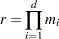Otherwise,is the number of cross-classifications actually observed.

If the function specification is MEANS, the number of response functions per population is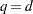.

### Response Statement Examples

Some example response statements are shown in the following table:

Example

Result

response marginals;

Marginals for each dependent variable

response means;

The mean of each dependent variable

response logits;

Generalized logits of the marginal probabilities

response clogits;

Cumulative logits of the marginal probabilities

response alogits;

Adjacent-category logits of the marginal probabilities

response joint;

The joint probabilities

response 1 -1 log;

The logit

response;

Generalized logits

response 1 2 3;

The mean score, with scores of 1, 2, and 3 corresponding to the three response levels

Four response functions and their covariance matrix, read directly from the input data set

### Using a Transformation to Specify Response Functions

If you specify a transformation, it is applied to the vector that contains the sample proportions in each population. The transformation can be any combination of the following four operations:

Operation

Specification

linear combinationmatrix literal

linear combination

matrix literal

logarithmexponentialmatrix literal

If more than one operation is specified, then PROC CATMOD applies the operations consecutively from right to left.

A matrix literal is a matrix of numbers with each row of the matrix separated from the next by a comma. If you specify a linear combination, in most cases theis not needed. The following statement defines the response function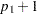. Theis needed to separate the two matrix literals '1' and '1 0'.

```response + 1 * 1 0;
```

Theof a vector transforms each element of the vector into its natural logarithm; theof a vector transforms each element into its exponential function (antilogarithm).

In order to specify a linear response function for data that have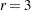response categories, you can specify either of the following RESPONSE statements:

```response  * 1 0 0 , 0 1 0;
response    1 0 0 , 0 1 0;
```

The matrix literal in the preceding statements specifies a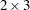matrix, which is applied to each population as follows: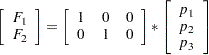where,, andare sample proportions for the three response categories in a population, and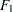and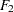are the two response functions computed for that population. Therefore, this response function sets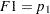and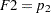in each population.

As another example of the linear response function, suppose you have two dependent variables corresponding to two observers who evaluate the same subjects. If the observers grade on the same three-point scale and if all nine possible responses are observed, then the following RESPONSE statement would compute the probability that the observers agree on their assessments:

```response 1 0 0 0 1 0 0 0 1;
```

This response function is then computed as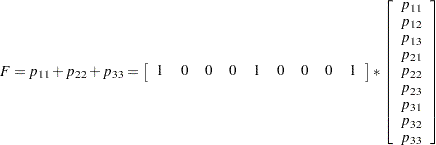where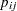denotes the probability that a subject gets a grade offrom the first observer andfrom the second observer.

If the function is a compound function, requiring more than one operation to specify it, then the operations should be listed so that the first operation to be applied is on the right and the last operation to be applied is on the left. For example, if there are two response levels, you can have the following response function:

```response 1 -1 log;
```

This is equivalent to the matrix expression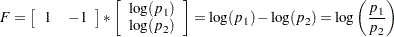which is the logit response function since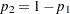when there are only two response levels.

The following statement specifies another example of a compound response function:

```response exp 1 -1 * 1 0 0 1, 0 1 1 0 log;
```

This is equivalent to the matrix expression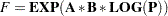where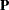is the vector of sample proportions for some population,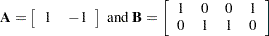If the four responses are based on two dependent variables, each with two levels, then the function can also be written aswhich is the odds (crossproduct) ratio for a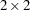table.

### Understanding the Standard Response Functions

If no RESPONSE statement is specified, PROC CATMOD computes the standard response functions, which contrast the log of each response probability with the log of the probability for the last response category. If there areresponse categories, then there are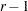standard response functions. For example, if there are four response categories, using no RESPONSE statement is equivalent to specifying the following:

```response  1 0 0 -1,
0 1 0 -1,
0 0 1 -1  log;
```

This results in three response functions: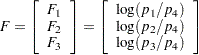If there are only two response levels, the resulting response function would be a logit, which is why the standard response functions are called generalized logits. They are useful in dealing with the log-linear model: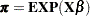If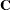denotes the matrix in the preceding RESPONSE statement, then because of the restriction that the probabilities sum to 1, it follows that an equivalent model is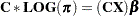But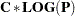is simply the vector of standard response functions. Thus, fitting a log-linear model on the cell probabilities is equivalent to fitting a linear model on the generalized logits.